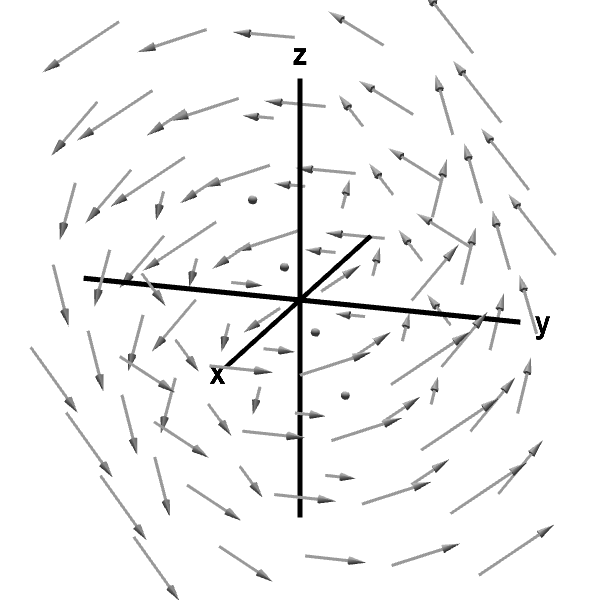# Math Insight

### Applet: A rotating vector fieldThis vector field illustrates rotation around an axis. The axis is halfway between the $x$ and $z$ axes. Along the axis of rotation, the vector field is zero. The zero vectors along this axis are displayed as dots. The rotation of the vector field gives it curl, which points parallel to the axis of rotation.

This vector field is $\dlvf(x,y,z)= (-y,x-z,y).$ Its curl is \begin{align*} \curl \dlvf(x,y,z) &= \left(\pdiff{}{y}y-\pdiff{}{z}(x-z), \pdiff{}{z}(-y)- \pdiff{}{x}y, \pdiff{}{x}(x-z) - \pdiff{}{y}(-y) \right)\\ &=(2,0,2), \end{align*} which is a constant independent of point $(x,y,z)$ and always points at an angle halfway between the positive $x$ and $z$ axes, parallel with the axis of rotation.

You can rotate the graph with your mouse to better visualize the three-dimensional perspective.